CAT  >  Test: Functions- 5

# Test: Functions- 5

Test Description

## 15 Questions MCQ Test Quantitative Aptitude (Quant) | Test: Functions- 5

Test: Functions- 5 for CAT 2022 is part of Quantitative Aptitude (Quant) preparation. The Test: Functions- 5 questions and answers have been prepared according to the CAT exam syllabus.The Test: Functions- 5 MCQs are made for CAT 2022 Exam. Find important definitions, questions, notes, meanings, examples, exercises, MCQs and online tests for Test: Functions- 5 below.
Solutions of Test: Functions- 5 questions in English are available as part of our Quantitative Aptitude (Quant) for CAT & Test: Functions- 5 solutions in Hindi for Quantitative Aptitude (Quant) course. Download more important topics, notes, lectures and mock test series for CAT Exam by signing up for free. Attempt Test: Functions- 5 | 15 questions in 15 minutes | Mock test for CAT preparation | Free important questions MCQ to study Quantitative Aptitude (Quant) for CAT Exam | Download free PDF with solutions
 1 Crore+ students have signed up on EduRev. Have you?
Test: Functions- 5 - Question 1

### The minimum value of ax2 + bx + c is 7/8 at x = 5/4. Find the value of the expression at x = 5, ifthe value of the expression at x = 1 is 1.

Detailed Solution for Test: Functions- 5 - Question 1

Correct Answer :- B

Explanation : ax2 + bx + c

a[x2 + b/a x + c/a]

a[x2 + 2b/2a x + c/a + (b/2a)2 - (b/2a)2]

a[(x+b/2a)2 + c/a - (b/2a)2]

Minimum value occurs when

x = - b/2a

=> -b/2a = 5/4

=> b/a = -5/2

And, minimum value is a[(c/a - (b/2a)2]

a[(c/a - (b/2a)2] = 7/8

=> c - 25s/16 = 7/8

=> 16c - 25a = 14.....(1)

Again value of the expression at x = 1 is 1

a * 12 + b * 1 + c = 1

a - 5a/2 + c = 1

=> 2a - 5a + 2c = 2

=> - 3a + 2c = 2.......(2)

Multiplying eq (2) by 8 and subtracting it from eq (1)

-a = -2

or a = 2

Thus, b = -5/2 × 2 = -5

Putting the value of a in eq (2)

-6 + 2c = 2

2c = 8

c = 4

Thus, the expressions is 2x2 - 5x + 4

Value of the expression at x = 5 is

2 * 52 - 5 * 5 + 4

= 50 - 25 + 4

= 29

Test: Functions- 5 - Question 2

### A function a(x) is defined for x as 3a(x) + 2a (2 – x) = (x + 3)2. What is the value of [G (–5)] where [x] represents the greatest integer less than or equal to x?

Detailed Solution for Test: Functions- 5 - Question 2

The equation given in the question is: 3a(x) + 2a (2–x) = (x + 3)2
Replacing x by (2–x) in the above equation, we get
3a(2–x) + 2a(x) = (5–x)2
Solving the above pairs of equation, we get
a(x) = (x2 + 38 – 23)/5
Thus, G(–5) = –188/5 = –37.6. The value of [–37.6] = –37. Hence, Option (c) is the correct

Test: Functions- 5 - Question 3

### Find the domain of the definition of the functiony = [(x – 3)/(x + 3)]1/2 + [(1 – x)/(1 + x)]1/2.

Detailed Solution for Test: Functions- 5 - Question 3

Both the brackets should be non-negative and neither (x + 3) nor (1+ x) should be 0.
For (x – 3)/(x + 3) to be non negative we have x>3 or x< – 3.
Also for (1– x)/(1+ x) to be non-negative –1 < x < 1. Since there is no interference in the two
ranges, Option (d) would be correct.

Test: Functions- 5 - Question 4

If f(x) = ex and g(x) = logex then value of fog will be

Detailed Solution for Test: Functions- 5 - Question 4

fog = f (logex) = elogex = x.

Test: Functions- 5 - Question 5

The function y = 1/x shifted 1 unit down and 1 unit right is given by

Detailed Solution for Test: Functions- 5 - Question 5

Looking at the options, one unit right means x is replaced by (x – 1). Also, 1 unit down means –1
on the RHS.
Thus, (y + 1) = 1/(x – 1)

Test: Functions- 5 - Question 6

If f(x) = |x – 2| , then which of the following is always true?

Detailed Solution for Test: Functions- 5 - Question 6

Take different values of n to check each option. Each of Options (a), (b) and (c) can be ruled out.
Hence, Option (d) is correct.

Test: Functions- 5 - Question 7

Read the instructions below and solve:
f(x) = f(x – 2) – f(x – 1), x is a natural number
f(1) = 0, f(2) = 1

Q.

The value of f[f(6)] is

Detailed Solution for Test: Functions- 5 - Question 7

f(1) = 0, f(2) = 1,
f(3) = f(1) – f(2) = –1
f(4) = f(2) – f(3) = 2
f(5) = f(3) – f(4) = –3
f(6) = f(4) – f(5) = 5
f(7) = f(5) – f(6) = –8
f(8) = f(6) – f(7) = 13

Ans:-

f(f(6) = f(5) = –3.

Test: Functions- 5 - Question 8

If f(x) is a function satisfying f(x). f(1/x) = f(x) + f(1/x) and f(4) = 65, what will be the value off(6)?

Detailed Solution for Test: Functions- 5 - Question 8

We have f(x) ◊ f(1/x) = f(x) + f(1/x)
fi f(1/x) [f(x) – 1] = f(x)
For x = 4, we have f(1/4) [f(4) – 1] = f (4)
fi f(1/4)  = 65
fi f(1/4) = 65/64 = 1/64 + 1
This means f(x) = x3 + 1
For f(6) we have f(6) = 216 + 1 = 217.

Test: Functions- 5 - Question 9

Define the functions:
A(x, y, z) = Max (max (x, y), min (y, z) min (x, z))
B(x, y, z) = Max (max (x, y), min (y, z) max (x, z))
C(x, y, z) = Max (min (x, y), min (y, z) min (x, z))
D(x, y, z) = Min (max (x, y), max (y, z) max (x, z))
Max (x, y, z) = Maximum of x, y and z.
Min (x, y, z) = Minimum of x, y and z.
Assume that x, y and z are distinct integers.

Q.

For what condition will A(x, y, z) be equal to Max (x, y, z)?

Detailed Solution for Test: Functions- 5 - Question 9

A(x, y, z) = Max (max (x, y), min (y, z) min (x, z))
B(x, y, z) = Max (max (x, y), min (y, z) max (x, z))
C(x, y, z) = Max (min (x, y), min (y, z) min (x, z))
D(x, y, z) = Min (max (x, y), max (y, z) max (x, z))
Max (x, y, z) = Maximum of x, y and z.
Min (x, y, z) = Minimum of x, y and z.
Assume that x, y and z are distinct integers.

Ans:-

When either x or y is maximum.

Test: Functions- 5 - Question 10

Define the functions:
A(x, y, z) = Max (max (x, y), min (y, z) min (x, z))
B(x, y, z) = Max (max (x, y), min (y, z) max (x, z))
C(x, y, z) = Max (min (x, y), min (y, z) min (x, z))
D(x, y, z) = Min (max (x, y), max (y, z) max (x, z))
Max (x, y, z) = Maximum of x, y and z.
Min (x, y, z) = Minimum of x, y and z.
Assume that x, y and z are distinct integers.

Q.

For what condition will A(x, y, z) not be equal to B (x, y, z)?

Detailed Solution for Test: Functions- 5 - Question 10

A(x, y, z) = Max (max (x, y), min (y, z) min (x, z))
B(x, y, z) = Max (max (x, y), min (y, z) max (x, z))
C(x, y, z) = Max (min (x, y), min (y, z) min (x, z))
D(x, y, z) = Min (max (x, y), max (y, z) max (x, z))
Max (x, y, z) = Maximum of x, y and z.
Min (x, y, z) = Minimum of x, y and z.
Assume that x, y and z are distinct integers.

Ans:-

When z is maximum, A and B would give different values. Thus, option (c) is correct.

Test: Functions- 5 - Question 11

Define the functions:
A(x, y, z) = Max (max (x, y), min (y, z) min (x, z))
B(x, y, z) = Max (max (x, y), min (y, z) max (x, z))
C(x, y, z) = Max (min (x, y), min (y, z) min (x, z))
D(x, y, z) = Min (max (x, y), max (y, z) max (x, z))
Max (x, y, z) = Maximum of x, y and z.
Min (x, y, z) = Minimum of x, y and z.
Assume that x, y and z are distinct integers.

Q.

The highest value amongst the following will be

Detailed Solution for Test: Functions- 5 - Question 11

A(x, y, z) = Max (max (x, y), min (y, z) min (x, z))
B(x, y, z) = Max (max (x, y), min (y, z) max (x, z))
C(x, y, z) = Max (min (x, y), min (y, z) min (x, z))
D(x, y, z) = Min (max (x, y), max (y, z) max (x, z))
Max (x, y, z) = Maximum of x, y and z.
Min (x, y, z) = Minimum of x, y and z.
Assume that x, y and z are distinct integers.

Ans:-

We cannot determine this because it would depend on whether the integers x, y, and z are positive
or negative.

Test: Functions- 5 - Question 12

A0, A1, A2,...... is a sequence of numbers withA0 = 1, A1 = 3, and At = (t +1) At–1 – t At–2 = 2, 3, 4,....Conclusion I. A8 = 77Conclusion II. A10 = 121Conclusion III. A12 = 145

Detailed Solution for Test: Functions- 5 - Question 12

This question is based on the logic of a chain function. Given the relationship
At = (t + 1) At – 1 – t At – 2
We can clearly see that the value of A2 would depend on the values of A0 and A1. Putting t = 2 in
the expression, we get:
A2 = 3A1 – 2A0 = 7; A3 = 19; A4 = 67 and A5 = 307. Clearly, A6 onwards will be larger than 307
and hence none of the three conclusions are true. Option (e) is the correct answer.

Test: Functions- 5 - Question 13

The figure below shows the graph of a function f (x). How many solutions does the equation f ( f(x)) = 15 have?

Detailed Solution for Test: Functions- 5 - Question 13

f ( f (x) = 15 when f (x) = 4 or f (x) = 12 in the given function. The graph given in the figure
becomes equal to 4 at 4 points and it becomes equal to 12 at 2 points in the figure. This gives us 6
points in the given figure when f (f (x) =15. However, the given function is continuous beyond the
part of it which is shown between –10 and +13 in the figure. Hence, we do not know how many
more solutions to f (f (x) = 15 would be there. Hence, Option (e) is the correct answer.

Test: Functions- 5 - Question 14

For all real numbers x, except x = 0 and x = 1, the function F is defined by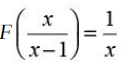If 0 < a < 90° then F((cosec a)2) =

Detailed Solution for Test: Functions- 5 - Question 14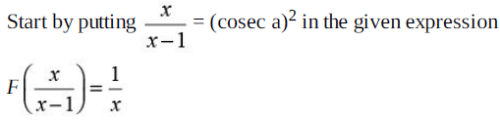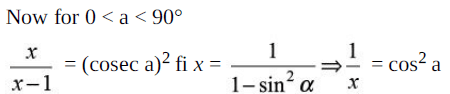Test: Functions- 5 - Question 15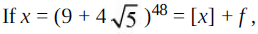where [x] is defined as integral part of x and f is a fraction, then x(1 – f) equals–

Detailed Solution for Test: Functions- 5 - Question 15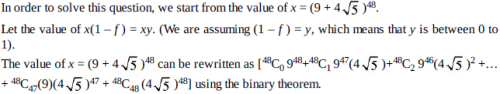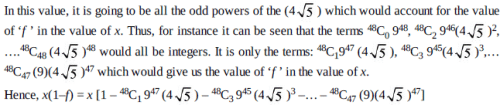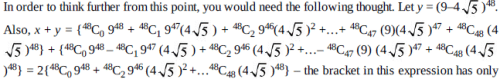retained the even terms which are integral. Hence, the value of x+y is an integer.
Further, x+y = [x] + f and hence, if x+y is an integer, [x] + f + y would also be an integer. This
automatically means that f+y must be an integer (as [x] is an integer).
Now, the value of y is between 0 to 1 and hence when we add the fractional part of x i.e. ‘f ’ to y,
and we need to make it an integer, the only possible integer that f + y can be equal to is 1.
Thus, if f + y = 1 Æ y = (1 – f ).
In order to find the value of x(1 – f ) we can find the value of x × y.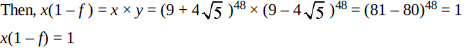## Quantitative Aptitude (Quant)

163 videos|152 docs|131 tests
 Use Code STAYHOME200 and get INR 200 additional OFF Use Coupon Code
Information about Test: Functions- 5 Page
In this test you can find the Exam questions for Test: Functions- 5 solved & explained in the simplest way possible. Besides giving Questions and answers for Test: Functions- 5, EduRev gives you an ample number of Online tests for practice

## Quantitative Aptitude (Quant)

163 videos|152 docs|131 tests

### How to Prepare for CAT

Read our guide to prepare for CAT which is created by Toppers & the best Teachers